#Existence and Uniqueness of SolutionsExistence and uniqueness theorem is the tool which makes it possible for us to conclude that there exists only one solution to a first order differential equation which satisfies a given initial condition. How does it work? Why is it the case? We believe it but it would be interesting to see the main ideas behind. First let us state the theorem itself.

Theorem. Let f(x,y) be a real valued function which is continuous on the rectangle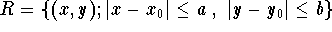. Assume f has a partial derivative with respect to y and that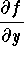is also continuous on the rectangle R. Then there exists an interval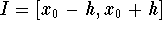(with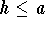) such that the initial value problem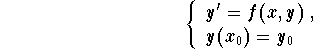has a unique solution y(x) defined on the interval I.

Note that the number h may be smaller than a. In order to understand the main ideas behind this theorem, assume the conclusion is true. Then if y(x) is a solution to the initial value problem, we must have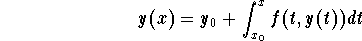It is not hard to see in fact that if a function y(x) satisfies the equation (called functional equation)on an interval I, then it is solution to the initial value problem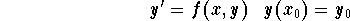Picard was among the first to look at the associated functional equation. The method he developed to find y is known as the method of successive approximations or Picard's iteration method. This is how it goes:

Step 1. Consider the constant function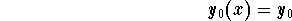Step 2. Once the function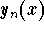is known, define the function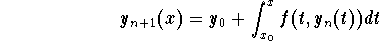Step 3. By induction, we generate a sequence of functions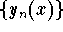which, under the assumptions made on f(x,y), converges to the solution y(x) of the initial value problemFor more on this, check the page Picard Iterative Process.

### If you would like to practice more, click on Example for more examples.[Differential Equations]
[Geometry] [Algebra] [Trigonometry ]
[Calculus] [Complex Variables] [Matrix Algebra]S.O.S MATHematics home page

Do you need more help? Please post your question on our S.O.S. Mathematics CyberBoard.Author: Mohamed Amine Khamsi